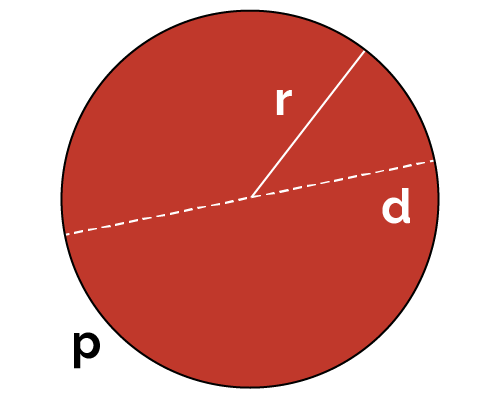# Circle Perimeter Calculator

Created by Luis Hoyos
Reviewed by Wojciech Sas, PhD candidate
Last updated: Sep 25, 2022

Our circle perimeter calculator can be of great help if you're struggling to solve geometry problems. One common problem is to find the perimeter of a circle, also called its circumference, which measures the length of the line forming this geometric figure. Keep reading if you want to know:

• What is the formula for circle perimeter.
• How to find the perimeter of a circle using the circle perimeter formula and our circle circumference calculator.
• What are the perimeters of a quarter circle and a half-circle.

🔎 Remember that, as with the rest of our tools, this circle circumference calculator works both ways - it is also a circumference to radius calculator - and you can use it to convert a circumference to a radius.

## The circle perimeter formula

If you don't know how to find the circumference of a circle, our calculator is the fastest option. Even so, it's helpful to understand how equations work to interpret the results better. The circle perimeter formula is:

p = 2πr,

where p is the circle's perimeter, r is the radius, and π is a constant equal to approximately 3.14159265...
You can also express the perimeter in terms of the diameter (d), remembering that r=d/2:

p = πd

💡 The number π is a constant equal to the ratio of the circumference to the diameter (or twice the radius). It'll always have the same value, independently of the size of the circle.
Solving for π in the above equation results in the mentioned ratio: π = p/(2r).

### Half circle and quarter circle perimeter formulas

Sometimes we need to calculate portions of a circle instead of a whole one. We can obtain the formulas for the perimeters of a half-circle and a quarter-circle by dividing the above equation by 2 and 4, respectively:

phalf = πr

pquarter = πr/2

## How to calculate the perimeter of a circle?

If you want to use the circle perimeter calculator to find the circumference of a circle, follow these steps:

1. Determine the radius of your circle. For example, let's suppose it's 7 cm.
2. Input the radius in the calculator, and you'll know the circle's circumference. The result should be 43.982 cm.
3. To double-check your result, enter the radius in the circle perimeter formula: p = 2 × π × (7 cm) = 43.982 cm.

## Other helpful tools beyond the circle perimeter calculator

Now that you know how to find the perimeter of a circle don't forget that the circumference to radius calculator is not our only tool dealing with circular objects. We're hopeful that the calculators below will help you with your day-to-day circular problems:

## FAQ

### How do I find the perimeter of a quarter circle?

To find the perimeter of a quarter circle:

1. Remember the perimeter of an ordinary circle, which is p = 2πr.
2. Divide it by four, as you only want the perimeter of a quarter circle.
3. The result should be p = πr/2. That is the perimeter of a quarter circle for any radius.

### How do I find the perimeter of a half-circle?

Multiply the radius by π (pi), and you'll get the half-circle perimeter. The perimeter of a half-circle is simply the perimeter of an ordinary circle divided by two, which results in p = πr.

### What is the perimeter of a 10-inch circle?

31.416 inches. To find the perimeter of any circle, multiply the diameter by the number pi (π). In this case, 10 in × π = 31.416 in.

Luis Hoyosin
Diameter (d)
in
Perimeter (p)
in
People also viewed…

### Distance between two points

This distance between two points calculator can instantly find the distance between two points on a two-dimensional plane!

### Flat vs. round Earth

Omni's not-flat Earth calculator helps you perform three experiments that prove the world is round.

### Millionaire

This millionaire calculator will help you determine how long it will take for you to reach a 7-figure saving or any financial goal you have. You can use this calculator even if you are just starting to save or even if you already have savings.

### Negative log

Use this online negative log calculator to calculate the negative logarithm of any number.